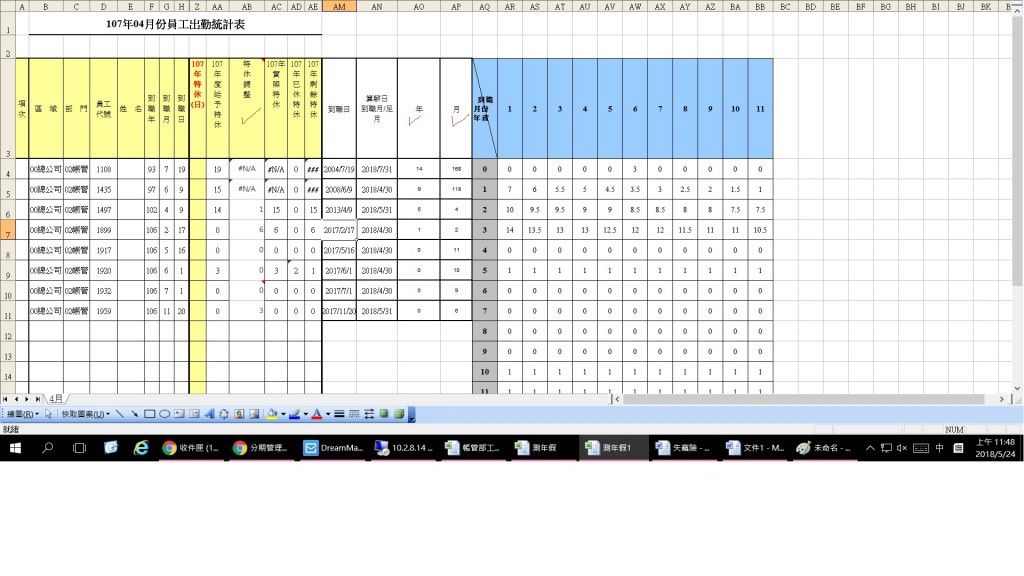0

excel 曆年特休問題

AN:算薪日/到職月 = 例六月，算五月薪水。會打2018/5/31。
AO:年 = AM- AN (=DATEDIF(AM4,AN4,"Y")) = 目前做了幾年。
AP:月 = 帶判斷 =
IF(OR(AO4=1, AO4=2AO4=3,AO4=5),G4,(DATEDIF(AM4,AN4,"M")))

AB(特休調整)寫法:
=IF(AND(AO4=0,AP4=6,),OR(AO4=0,AO4=1,AO4=2,AO4=3,AO4=5),INDEX(\$AR\$4:\$BB\$101,MATCH(\$AO4,\$AQ\$4:\$AQ\$101),MATCH(\$AP4,\$AR\$3:\$BB\$3,0)))swhung iT邦新手 5 級 ‧ 2018-05-25 09:47:46 檢舉